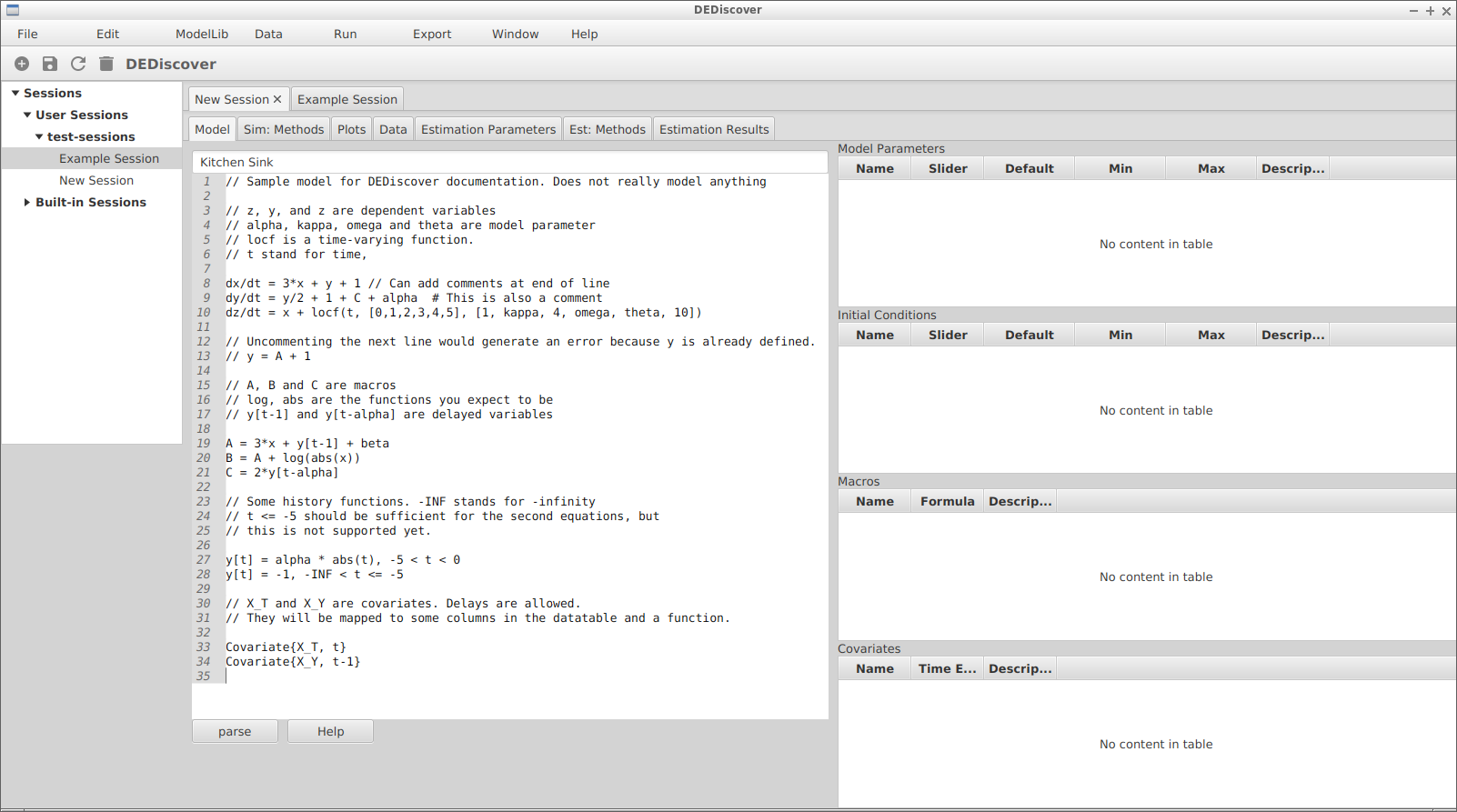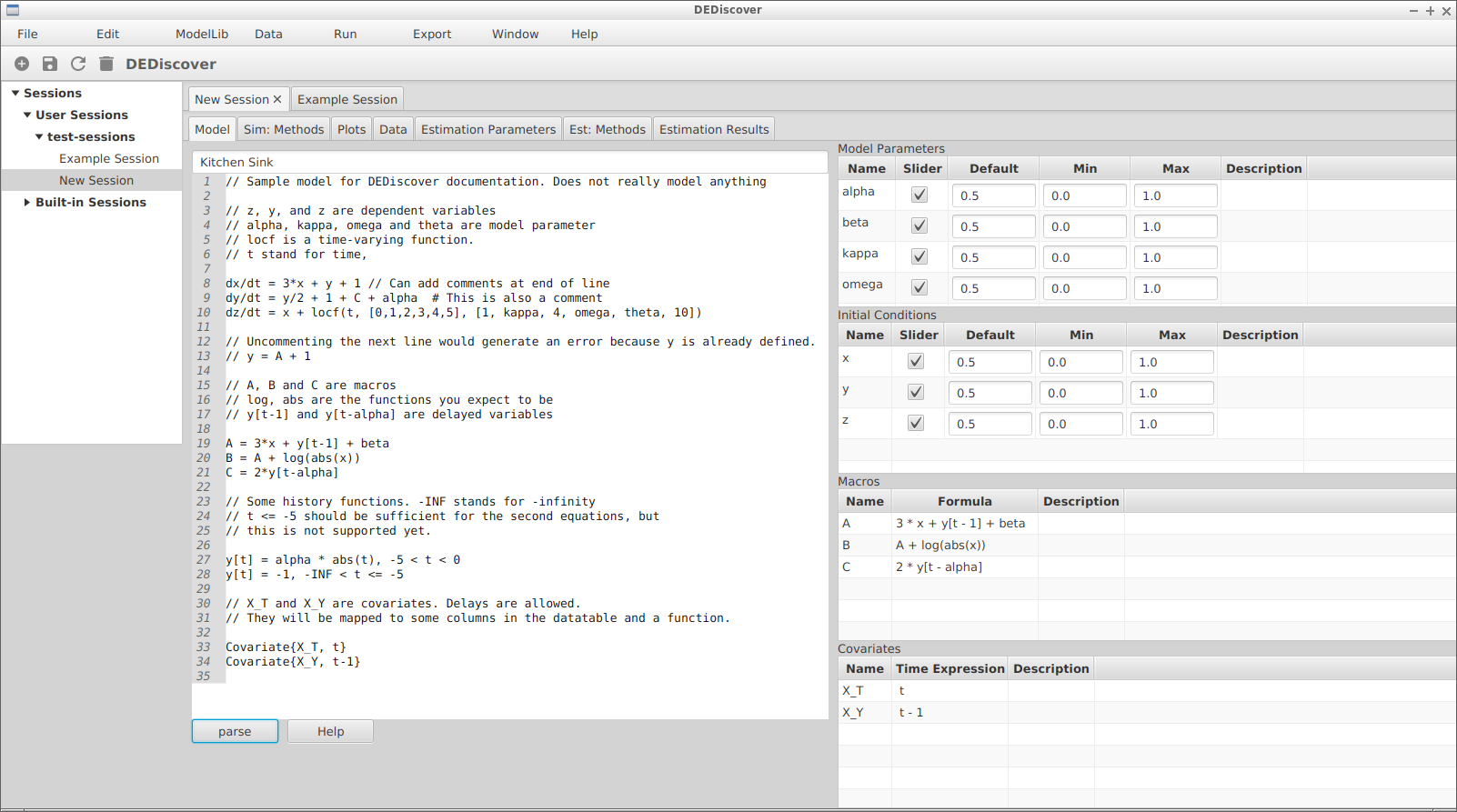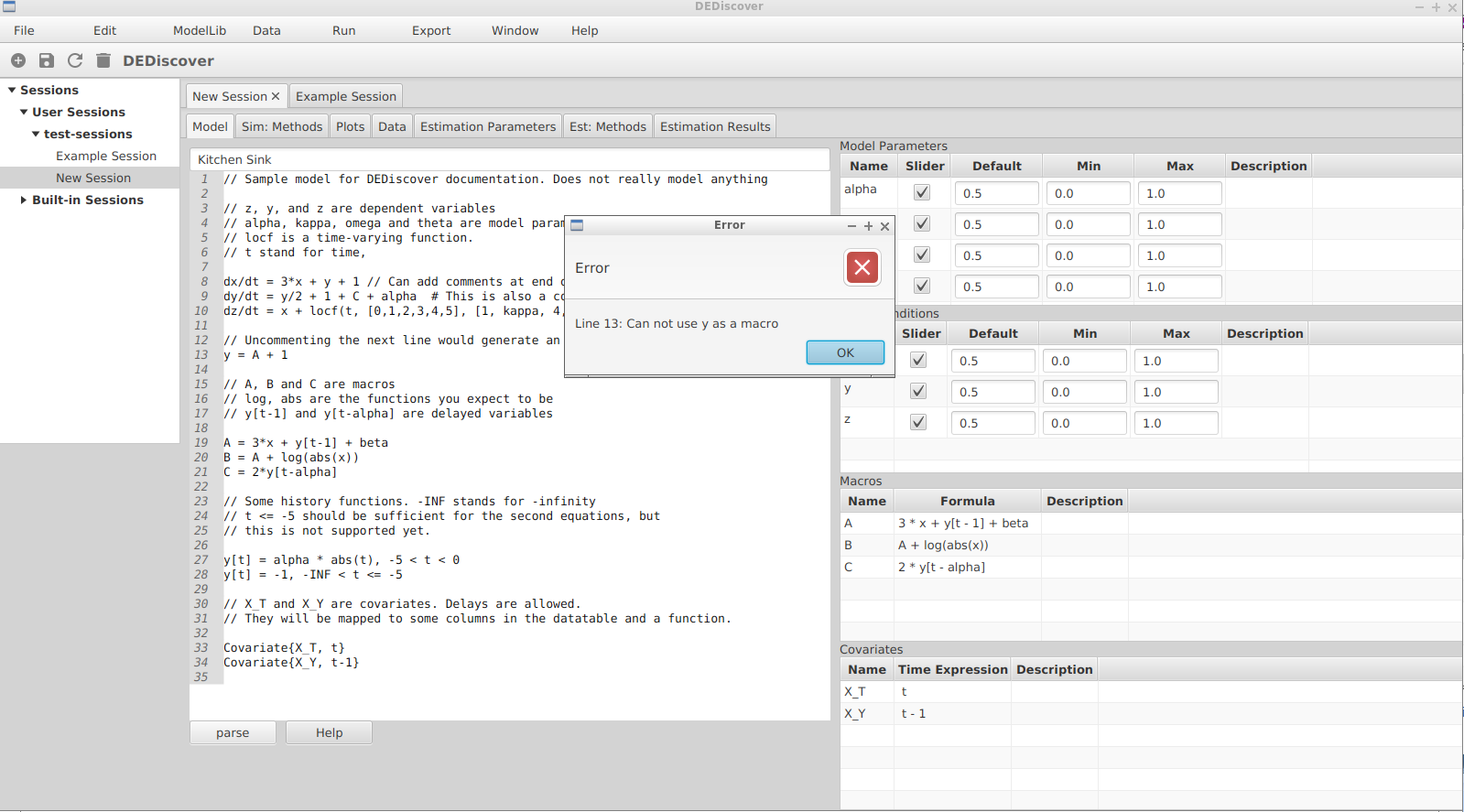# Creating a new model in DEDiscover¶

## Writing the model¶

A user creates a new session with the Plus button in the button bar, by selecting File -> New Session from the top menu, or right-clicking on a package in the session tree. Then the model defined by the equations can be entered in the editor window. The figure below shows such a model. It does not describe any real life system, but it illustrates many of the features that are supported.A new session with equations

A detailed description of the syntax is found in the model syntax page, but for now we we will give a overview of the different types of equations that are recognized by DEDiscover, along with some basic rules and conventions.

1. The equations are written on a line by line basis. That is, an equation can not span multiple lines. If it needs to be longer than the width of the window then it will wrap, but it is still consider one line. Also a line terminated by a slash (\) indicates that the next line is a continuation of the current line.

2. All the contents following two forward slashes (//) or a hashtag (#) define comments that go to the end of the line.

3. Any line of the form

dVarName/dt = …

is a differential equation, where VarName stands for any legal symbol, as described in the model syntax. Lines 8, 9 and 10 are differential equations, and so x, y and z are dependent variables.

4. Any line of the form

macroName = ….

is a macro, where macroName is any legal symbol. Lines 19, 20 and 21 define macros A, B and C.

5. Lines 27 and 28 define history functions. They are used in a model with delay variables to obtain the values for the dependent variable at times occurring before the beginning of the simulation. In this model dependent variable y is a delay variable because of the way it is used in lines 19 and 21. If no history function is defined for a given time then its value defaults to 0. If there is overlap then the value for one of the intervals will be used, but it is undefined which one it will be.

6. Lines 33 and 34 define covariates. More details at the model syntax page.

7. Any symbol that is not a dependent variable, a macro, a covariate, or function, or any other reserved word is a model parameter. In the model above alpha, beta, kappa, omega and theta are model parameters.

## Validating and compiling the model¶

After the equations have been entered they must be parsed, or compiled, by DEDiscover to ensure that the syntax is correct. If there are no errors then DEDiscover will identify all the different components, such as the differential equations, macros, dependent variables, model parameters, covariates and history functions. At this point the right hand side of the model tab is populated with the appropriate values, and the user can add information about the different symbols, such as minimum, maximum and default values, and add a description of the variable or parameter.If we were to uncomment line 13 and click on parse again, DEDiscover would notice that y is defined as both a macro and a dependent variable, and issue a more or less understandable error message. Then no other operation for this session would be allowed until the model has been corrected and parsed. The figure below shows the message.